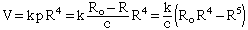Hi there. My name is Ena, and I am currently studying a maths & stats class at university. Last week we were given the following problems & I cannot figure them out, I was wondering if you could help me in anyway at all: Q1. The volume of air flowing in windpipes is given by V=kpR4, where k is a constant, p is the pressure difference at each end, R is the radius. The radius will decrease with increased pressure, according to the formula: Ro - R = cp, where Ro is the windpipe radius when p=0 & c is a positive constant. R is restricted such that: 0 < 0.5*Ro < R < Ro, find the factor by which the radius of the windpipe contracts to give maximum flow? Q2. Reaction R to a dose x is given by: R= 5.3lnx + x For a certain drug, R must not exceed 21. Show that a dose between 8 and 12 units satisfies this requirement & find, correct to 5 decimal places, the greates value of x which satisfies this condition? Q3. If D'(t) = 100e4.32t, find the total increase during the first 3 days? I hope that you can offer me advice on obtaining solutions, or worked answers, or solutions themselves! Thank you, it is much appreciated! Ena Hi Ena, Let me get you started on the first question. You have V = k p R4 Where k is a constant and both p and R are changing. There is, however, a relationship between p and R, Ro - R = c p Here Ro and c are constants. Thus p = (Ro - R)/c and thusYou are to maximize V so find dV/dR, set it equal to zero and solve for R. Make sure that the value of R you find satisfies 0 < 0.5*Ro < R < Roand that it produces a maximum. Cheers, Harley Go to Math Central